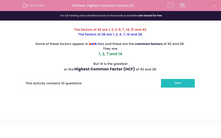# Find the Highest Common Factor of Larger Numbers

In this worksheet, children will be asked to state the highest common factor of two numbers.Key stage:  KS 2

Curriculum topic:   Maths and Numerical Reasoning

Curriculum subtopic:   Factors and Multiples

Difficulty level:#### Worksheet Overview

The factors of 42 are 1, 2, 3, 6, 7, 14, 21 and 42.

The factors of 28 are 1, 2, 4, 7, 14 and 28.

Some of these factors appear in both lists and these are the common factors of 42 and 28.

They are: 1, 2, 7 and 14

But 14 is the greatest or the highest common factor (HCF) of 42 and 28.

Let's have a go at some questions now.

### What is EdPlace?

We're your National Curriculum aligned online education content provider helping each child succeed in English, maths and science from year 1 to GCSE. With an EdPlace account you’ll be able to track and measure progress, helping each child achieve their best. We build confidence and attainment by personalising each child’s learning at a level that suits them.

Get started